# Selina Solutions Concise Mathematics Class 6 Chapter 25 Properties of Angles and Lines

Selina Solutions Concise Mathematics Class 6 Chapter 25 Properties of Angles and Lines are well structured by highly experienced teachers at BYJU’S, as per the understanding capacity of students. The solutions help students, to improve confidence and solve the problems with ease in the annual exam. Practising these solutions, on a daily basis, enable students to obtain expertise in Mathematics. Students who aspire to secure high marks, can refer to Selina Solutions Concise Mathematics Class 6 Chapter 25 Properties of Angles and Lines PDF links, which are given here with free download option.

## Selina Solutions Concise Mathematics Class 6 Chapter 25: Properties of Angles and Lines Download PDF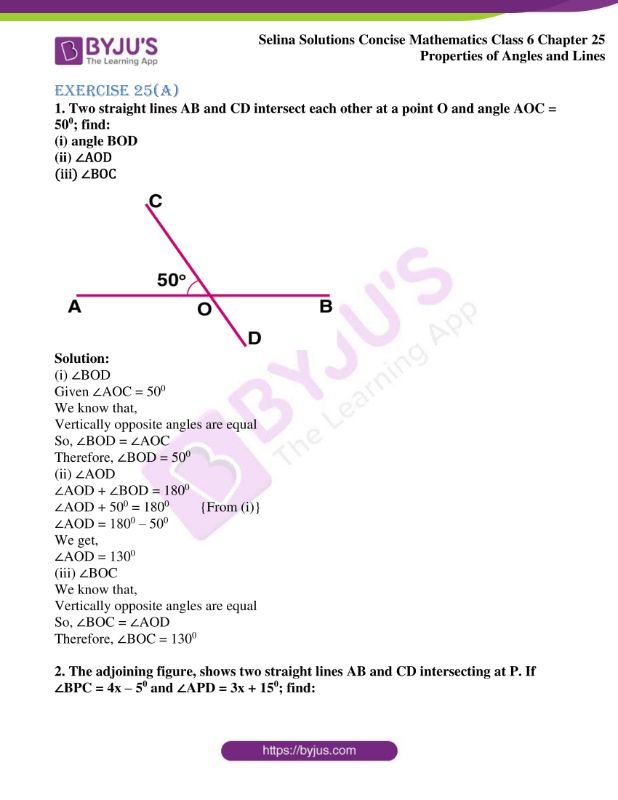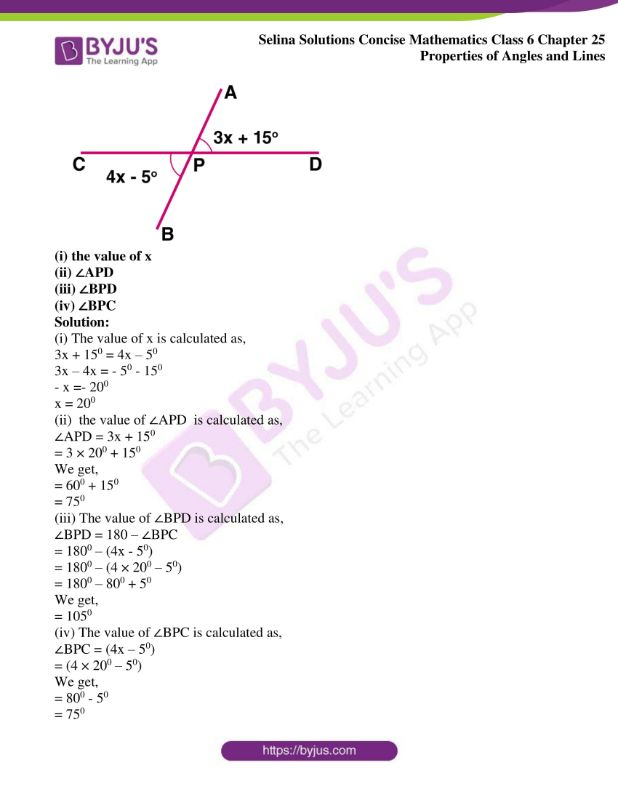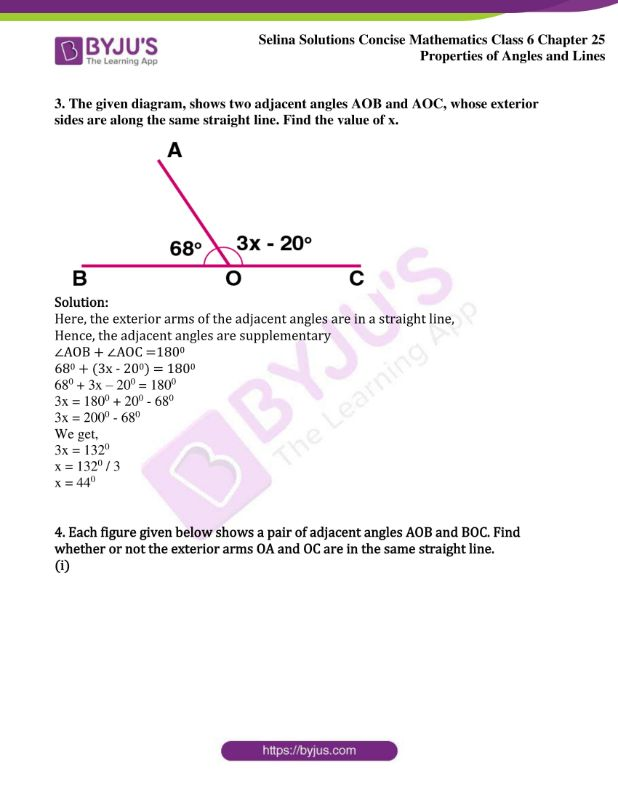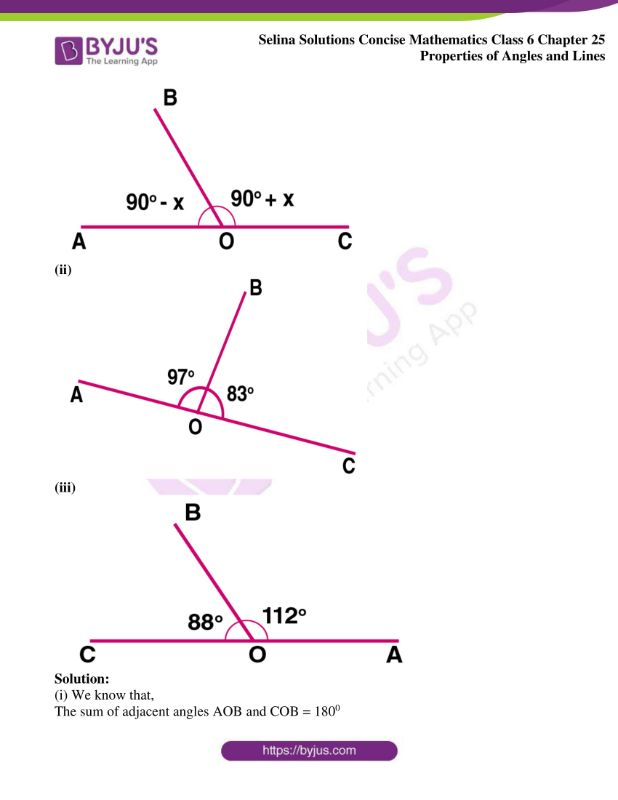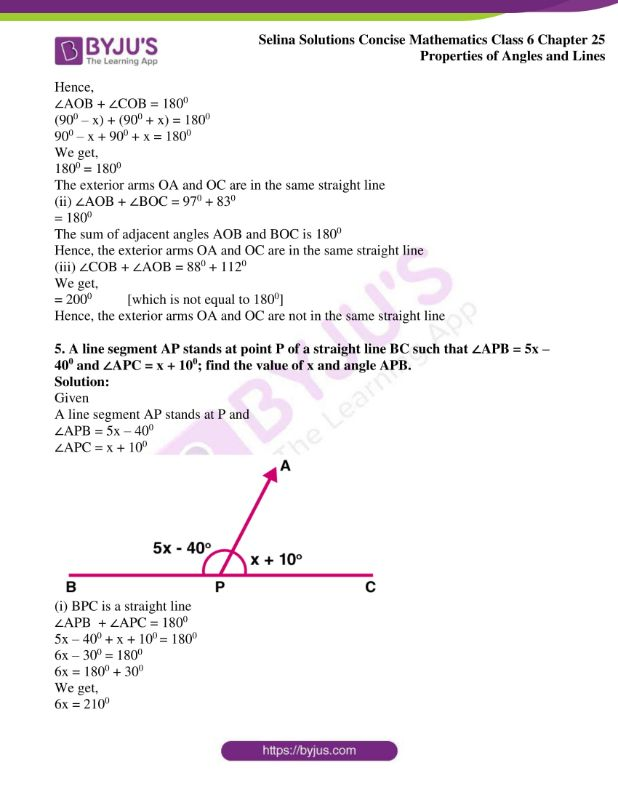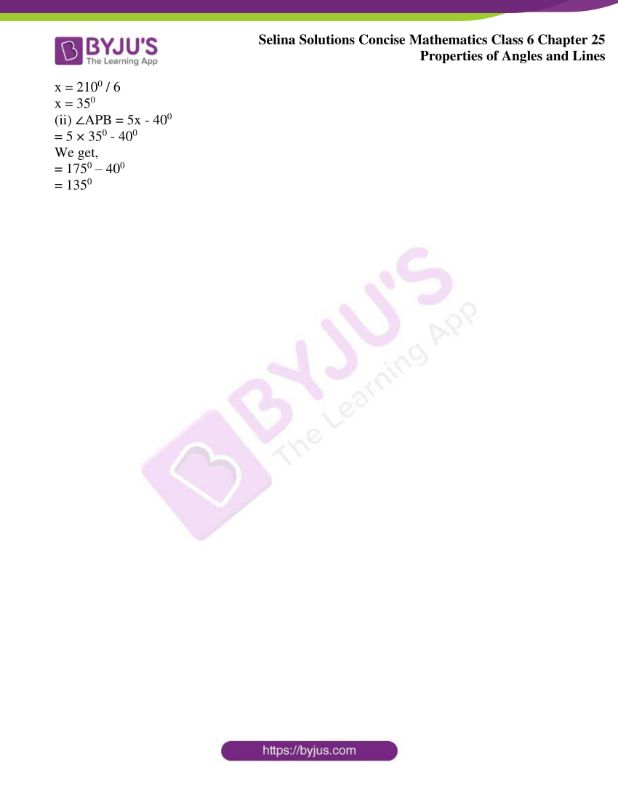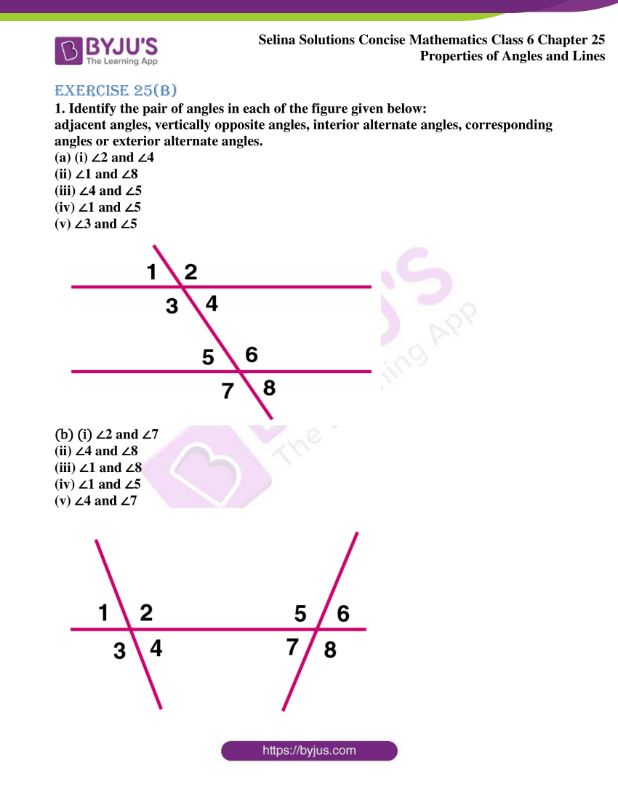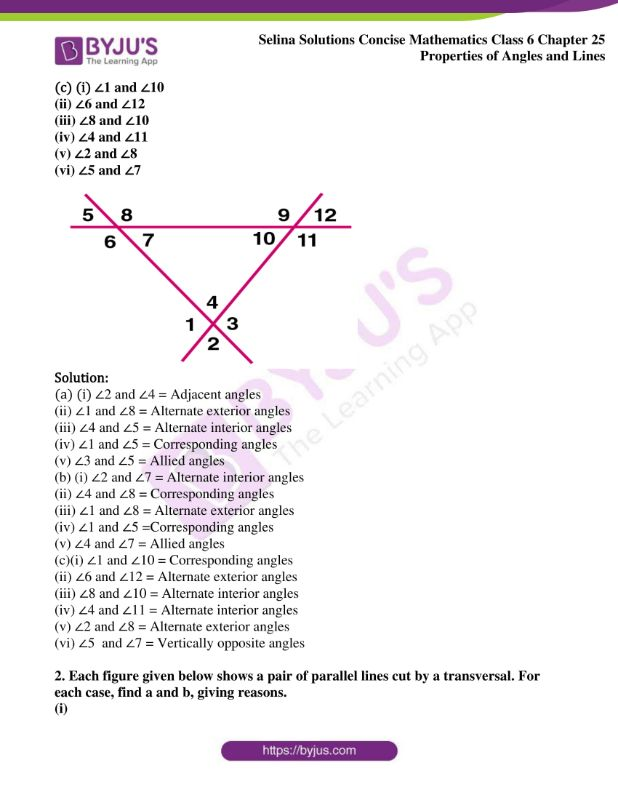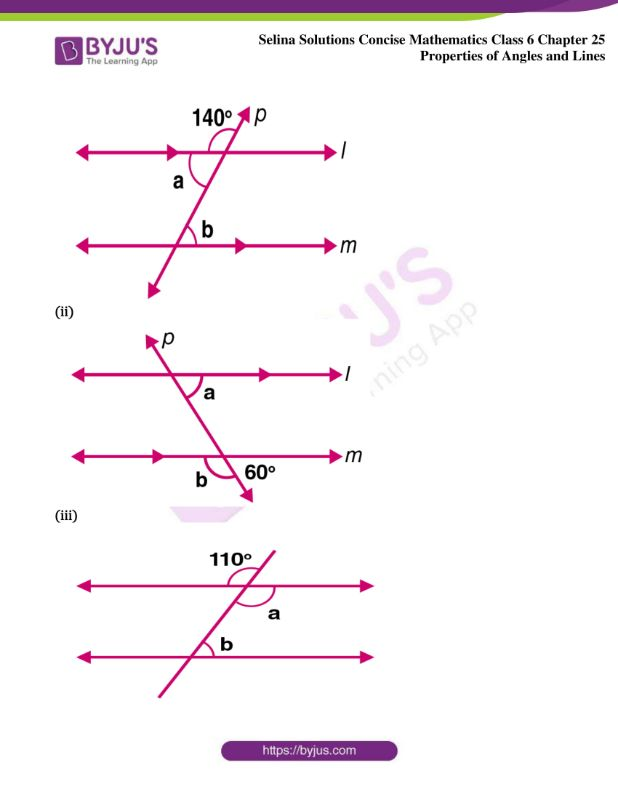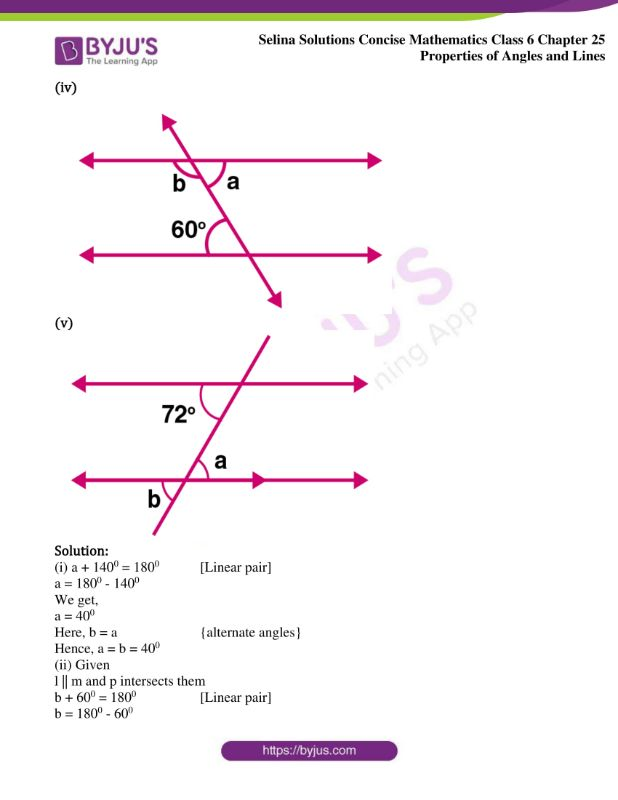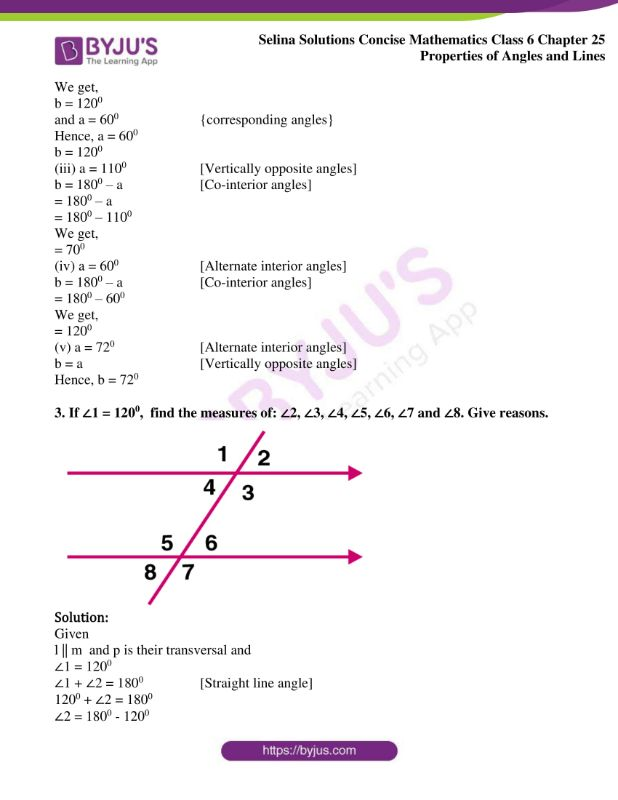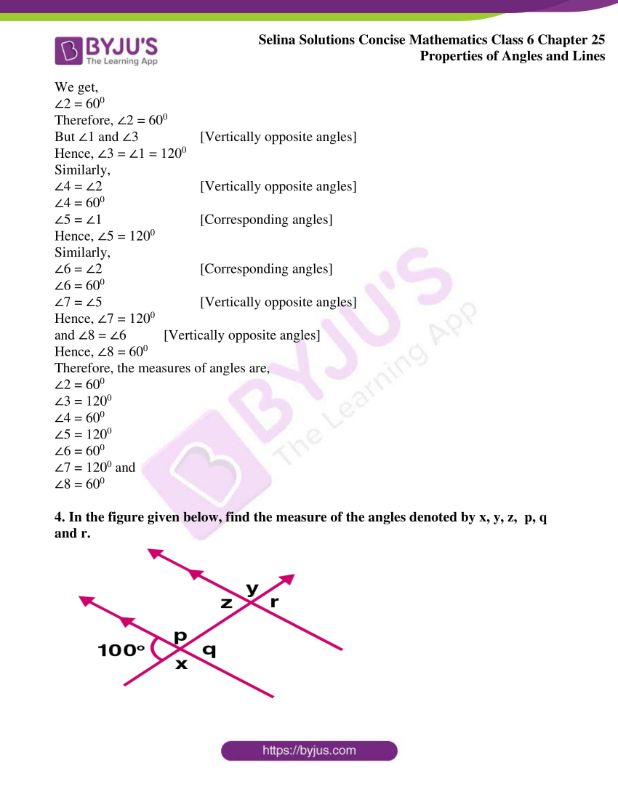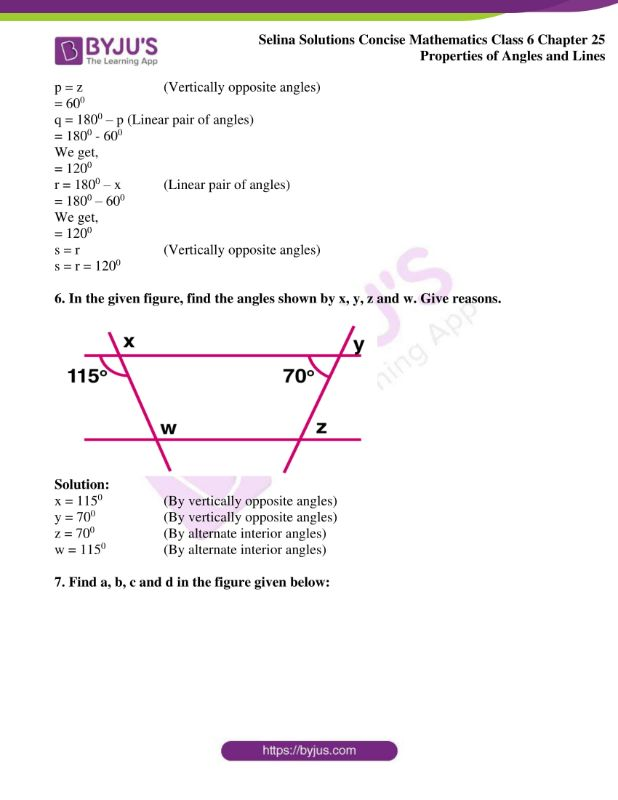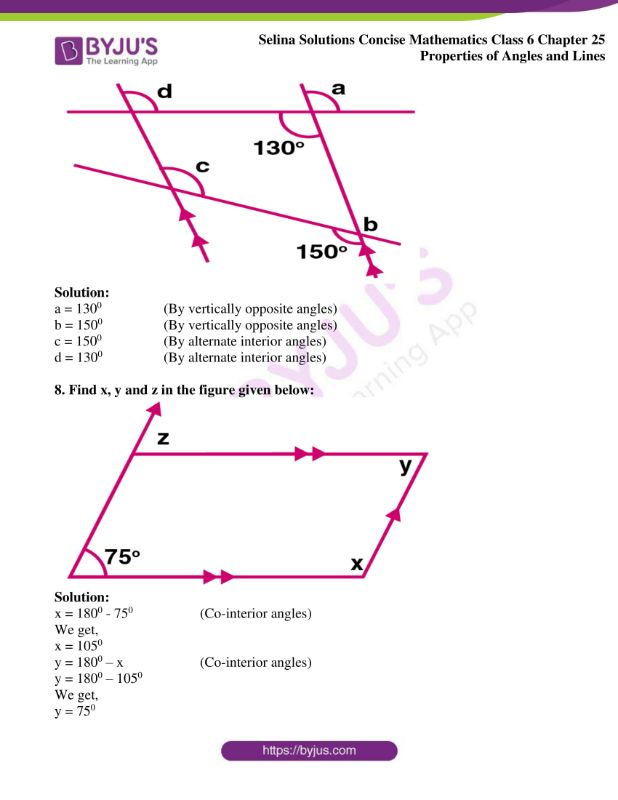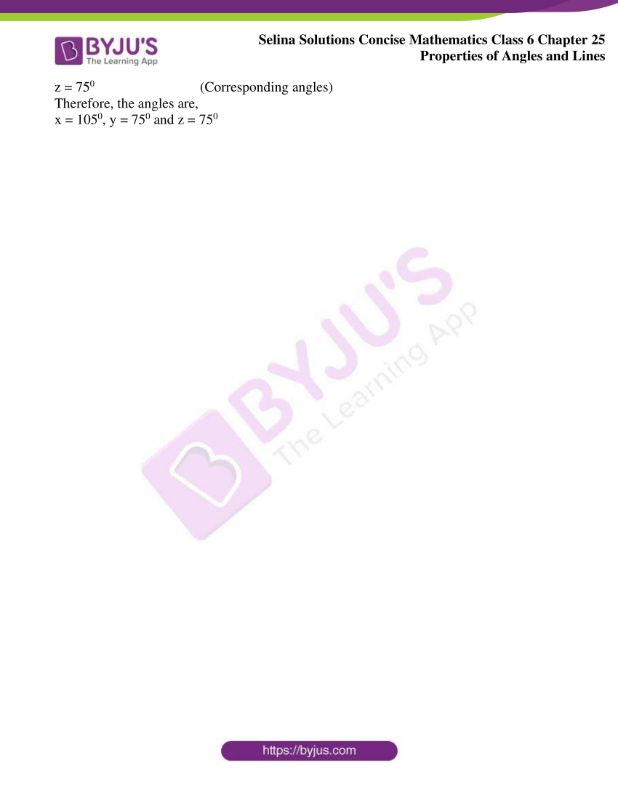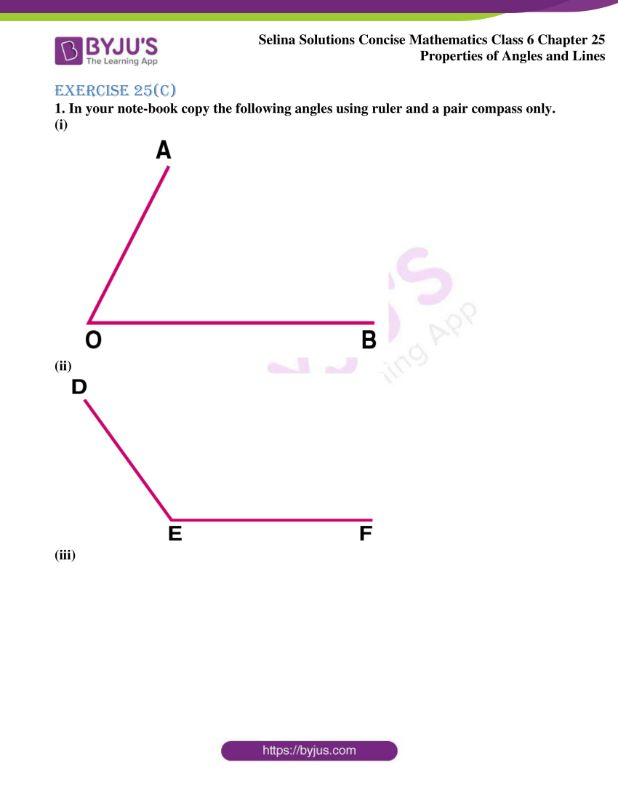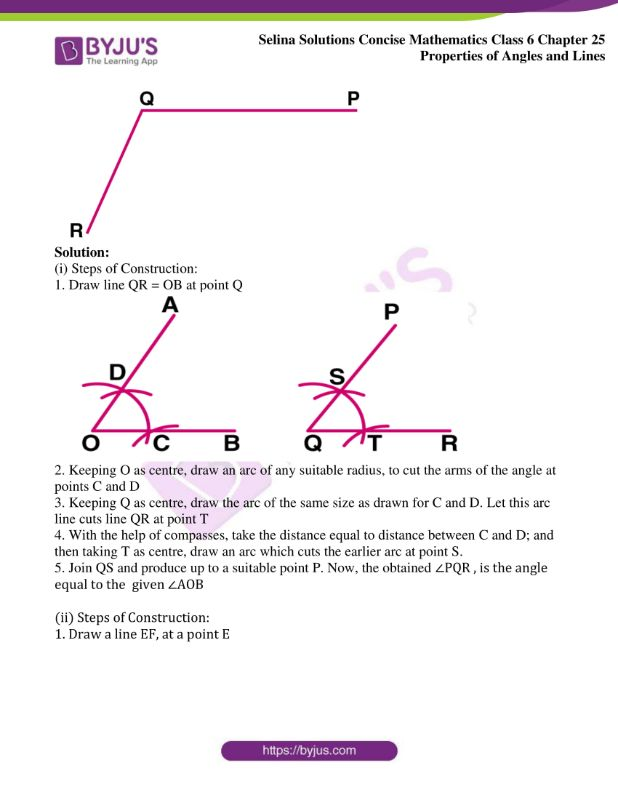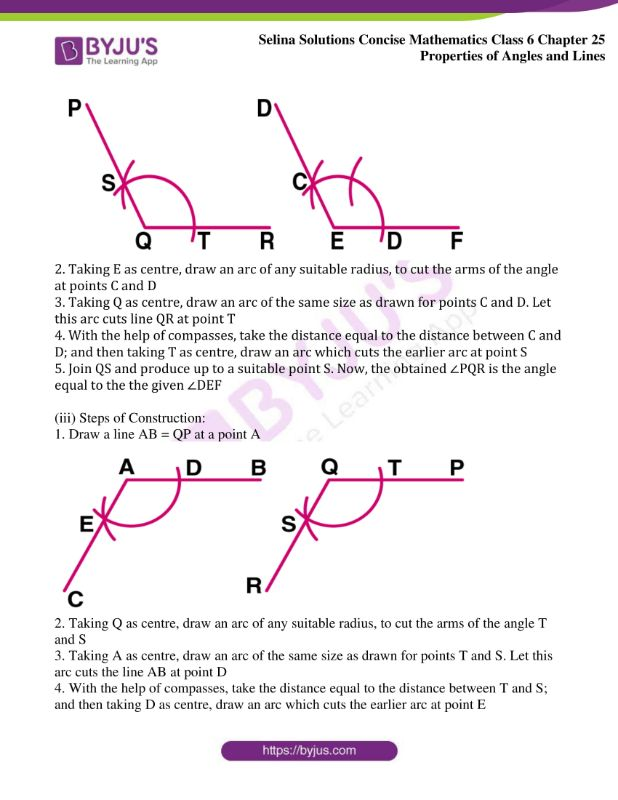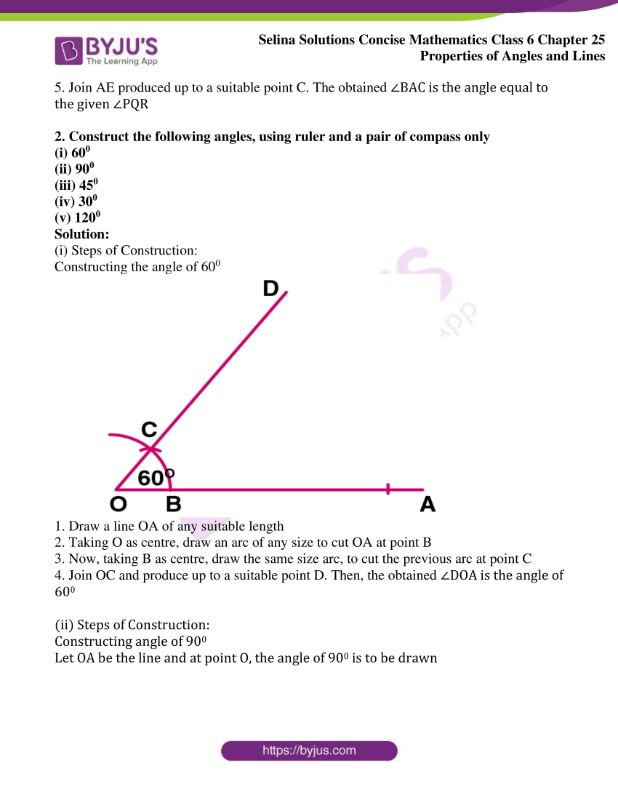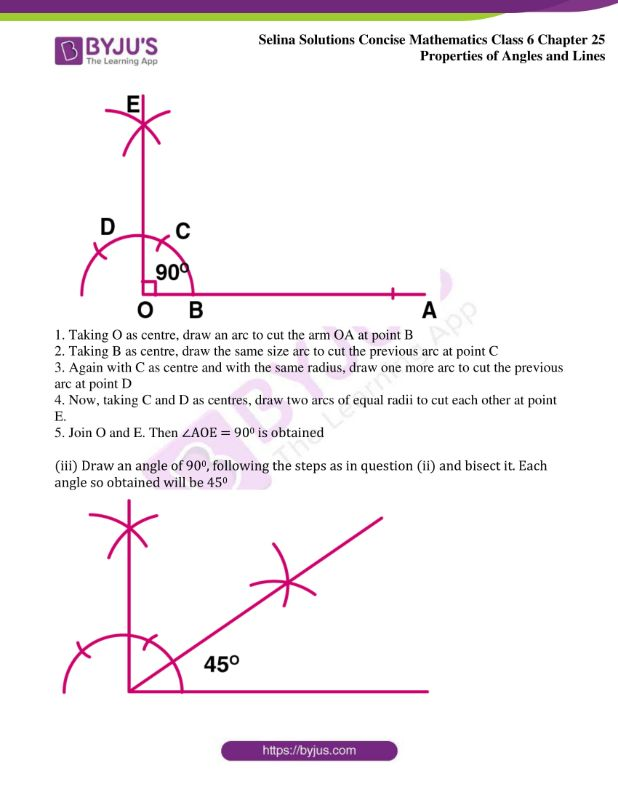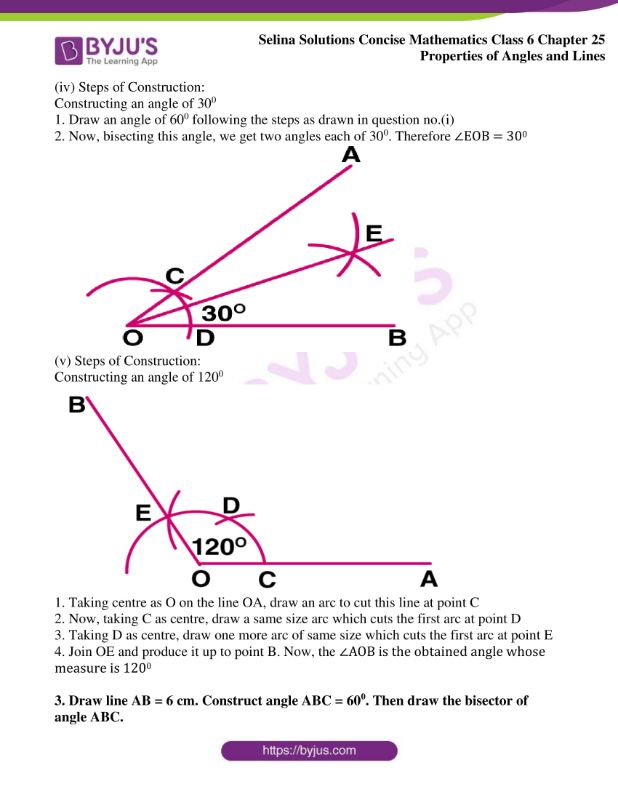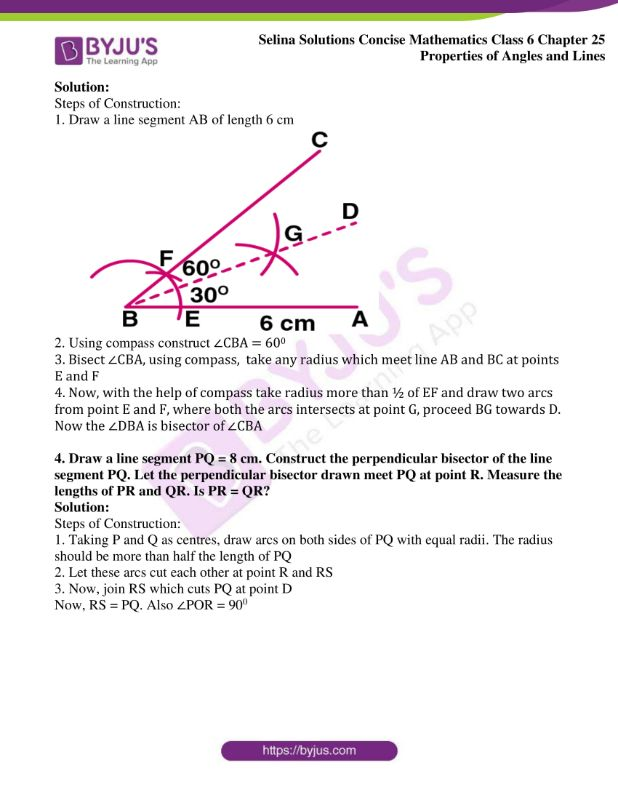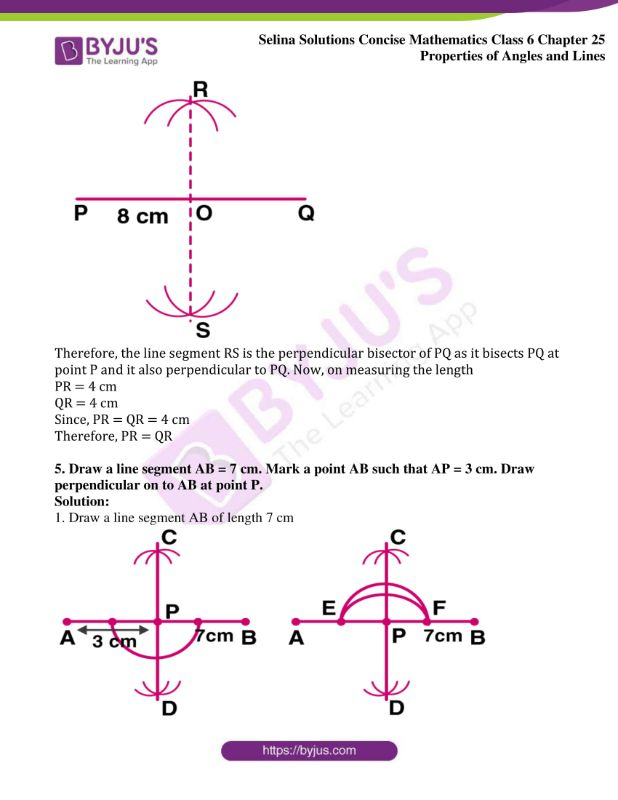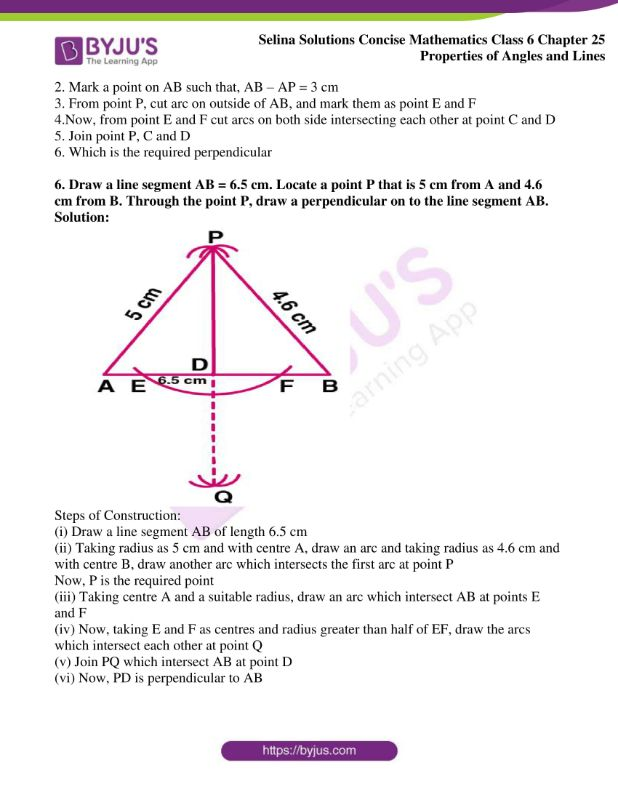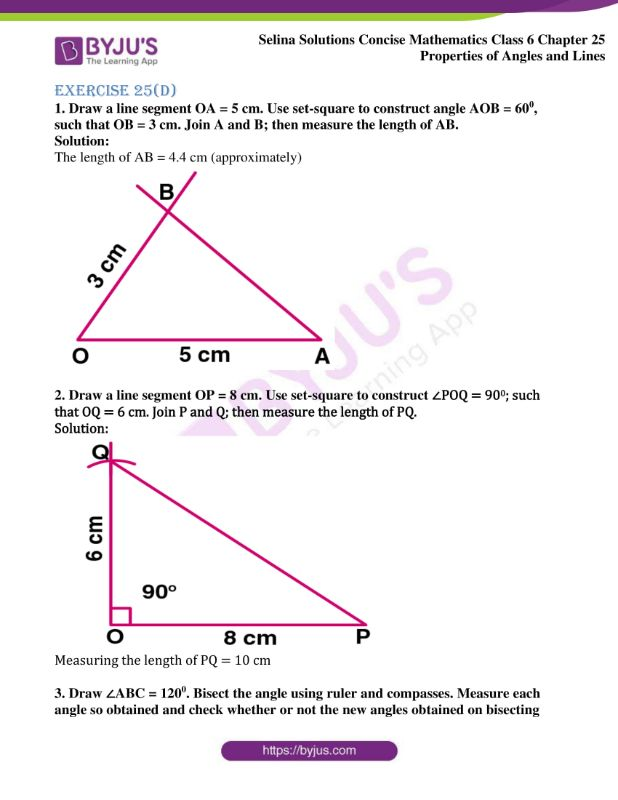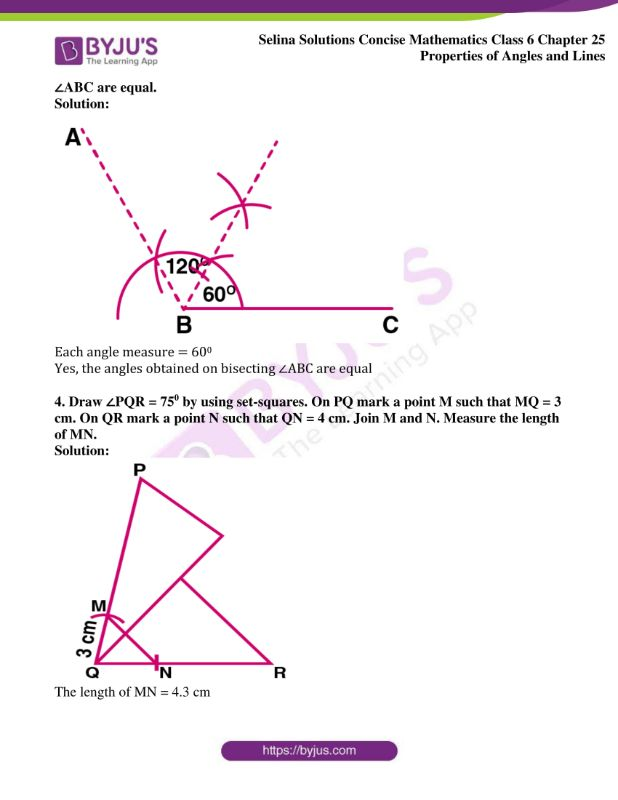## Access Selina Solutions Concise Mathematics Class 6 Chapter 25: Properties of Angles and Lines

Exercise 25(A)

1. Two straight lines AB and CD intersect each other at a point O and angle AOC = 500; find:

(i) angle BOD

(ii) ∠AOD

(iii) ∠BOC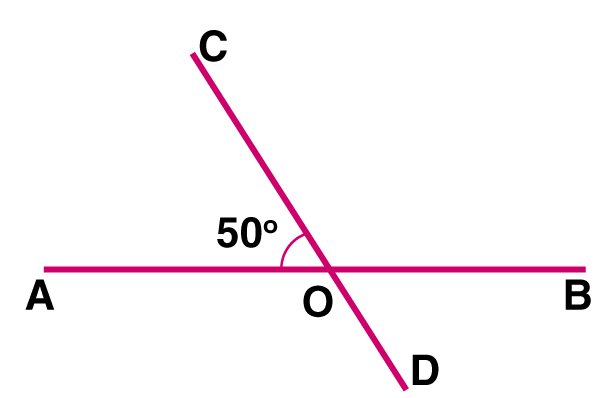Solution:

(i) ∠BOD

Given ∠AOC = 500

We know that,

Vertically opposite angles are equal

So, ∠BOD = ∠AOC

Therefore, ∠BOD = 500

(ii) ∠AOD

∠AOD + ∠BOD = 1800

∠AOD + 500 = 1800 {From (i)}

∠AOD = 1800 – 500

We get,

∠AOD = 1300

(iii) ∠BOC

We know that,

Vertically opposite angles are equal

So, ∠BOC = ∠AOD

Therefore, ∠BOC = 1300

2. The adjoining figure, shows two straight lines AB and CD intersecting at P. If ∠BPC = 4x – 50 and ∠APD = 3x + 150; find: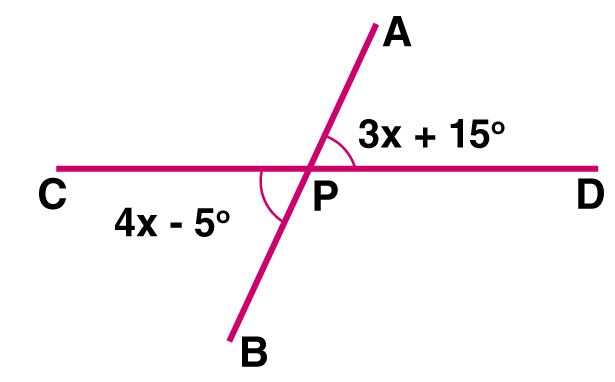(i) the value of x

(ii) ∠APD

(iii) ∠BPD

(iv) ∠BPC

Solution:

(i) The value of x is calculated as,

3x + 150 = 4x – 50

3x – 4x = – 50 – 150

– x =- 200

x = 200

(ii) the value of ∠APD is calculated as,

∠APD = 3x + 150

= 3 × 200 + 150

We get,

= 600 + 150

= 750

(iii) The value of ∠BPD is calculated as,

∠BPD = 180 – ∠BPC

= 1800 – (4x – 50)

= 1800 – (4 × 200 – 50)

= 1800 – 800 + 50

We get,

= 1050

(iv) The value of ∠BPC is calculated as,

∠BPC = (4x – 50)

= (4 × 200 – 50)

We get,

= 800 – 50

= 750

3. The given diagram, shows two adjacent angles AOB and AOC, whose exterior sides are along the same straight line. Find the value of x.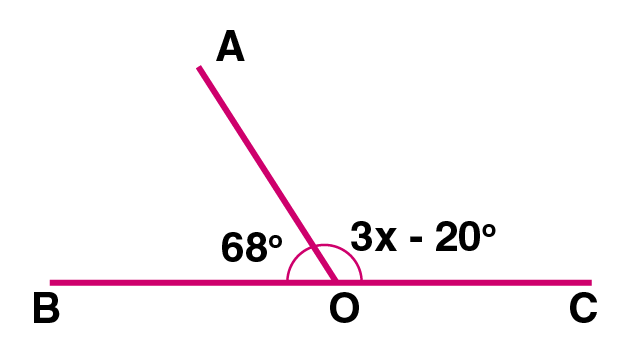Solution:

Here, the exterior arms of the adjacent angles are in a straight line,

Hence, the adjacent angles are supplementary

∠AOB + ∠AOC =1800

680 + (3x – 200) = 1800

680 + 3x – 200 = 1800

3x = 1800 + 200 – 680

3x = 2000 – 680

We get,

3x = 1320

x = 1320 / 3

x = 440

4. Each figure given below shows a pair of adjacent angles AOB and BOC. Find whether or not the exterior arms OA and OC are in the same straight line.

(i)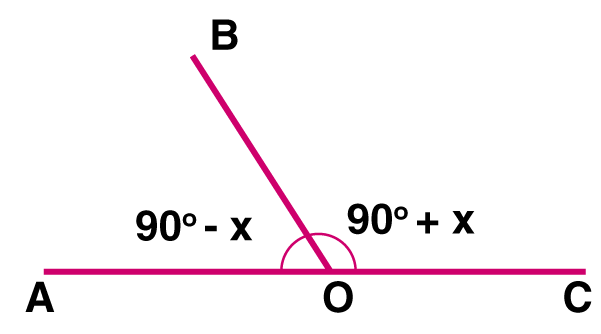(ii)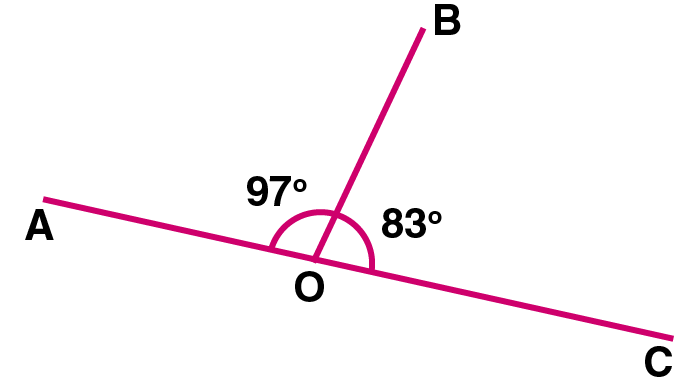(iii)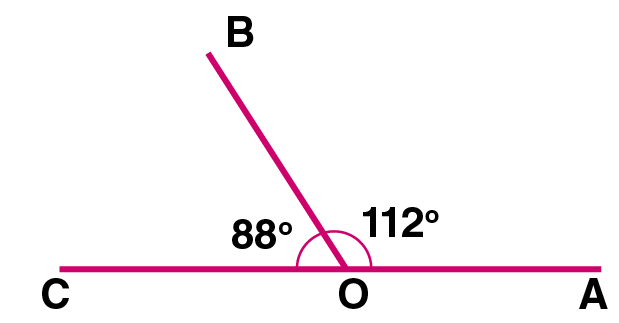Solution:

(i) We know that,

The sum of adjacent angles AOB and COB = 1800

Hence,

∠AOB + ∠COB = 1800

(900 – x) + (900 + x) = 1800

900 – x + 900 + x = 1800

We get,

1800 = 1800

The exterior arms OA and OC are in the same straight line

(ii) ∠AOB + ∠BOC = 970 + 830

= 1800

The sum of adjacent angles AOB and BOC is 1800

Hence, the exterior arms OA and OC are in the same straight line

(iii) ∠COB + ∠AOB = 880 + 1120

We get,

= 2000 [which is not equal to 1800]

Hence, the exterior arms OA and OC are not in the same straight line

5. A line segment AP stands at point P of a straight line BC such that ∠APB = 5x – 400 and ∠APC = x + 100; find the value of x and angle APB.

Solution:

Given

A line segment AP stands at P and

∠APB = 5x – 400

∠APC = x + 100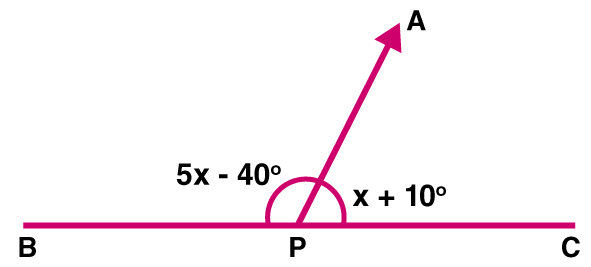(i) BPC is a straight line

∠APB + ∠APC = 1800

5x – 400 + x + 100 = 1800

6x – 300 = 1800

6x = 1800 + 300

We get,

6x = 2100

x = 2100 / 6

x = 350

(ii) ∠APB = 5x – 400

= 5 × 350 – 400

We get,

= 1750 – 400

= 1350

Exercise 25(B)

1. Identify the pair of angles in each of the figure given below:

adjacent angles, vertically opposite angles, interior alternate angles, corresponding angles or exterior alternate angles.

(a) (i) ∠2 and ∠4

(ii) ∠1 and ∠8

(iii) ∠4 and ∠5

(iv) ∠1 and ∠5

(v) ∠3 and ∠5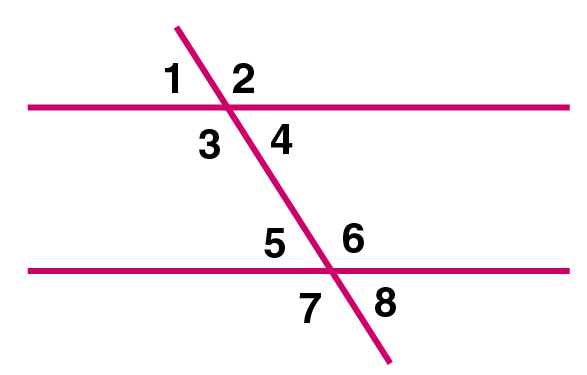(b) (i) ∠2 and ∠7

(ii) ∠4 and ∠8

(iii) ∠1 and ∠8

(iv) ∠1 and ∠5

(v) ∠4 and ∠7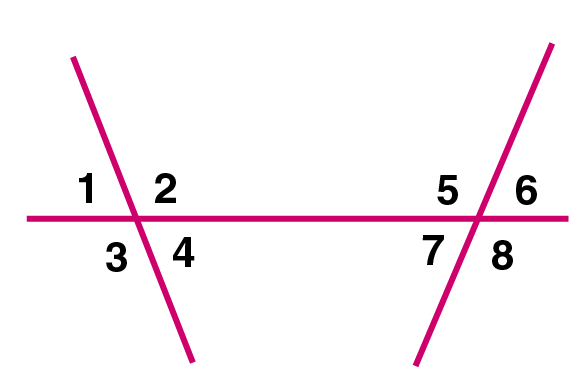(c) (i) ∠1 and ∠10

(ii) ∠6 and ∠12

(iii) ∠8 and ∠10

(iv) ∠4 and ∠11

(v) ∠2 and ∠8

(vi) ∠5 and ∠7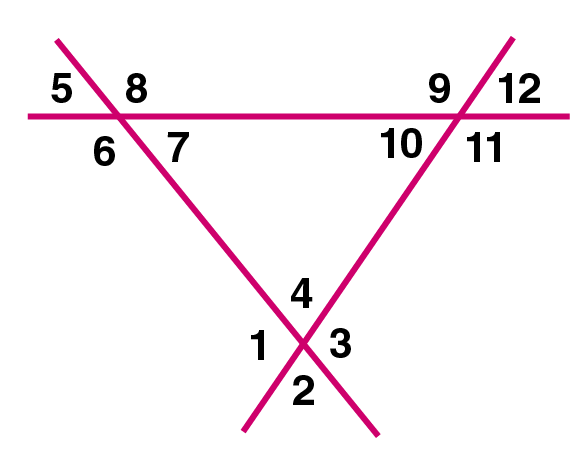Solution:

(a) (i) ∠2 and ∠4 = Adjacent angles

(ii) ∠1 and ∠8 = Alternate exterior angles

(iii) ∠4 and ∠5 = Alternate interior angles

(iv) ∠1 and ∠5 = Corresponding angles

(v) ∠3 and ∠5 = Allied angles

(b) (i) ∠2 and ∠7 = Alternate interior angles

(ii) ∠4 and ∠8 = Corresponding angles

(iii) ∠1 and ∠8 = Alternate exterior angles

(iv) ∠1 and ∠5 =Corresponding angles

(v) ∠4 and ∠7 = Allied angles

(c)(i) ∠1 and ∠10 = Corresponding angles

(ii) ∠6 and ∠12 = Alternate exterior angles

(iii) ∠8 and ∠10 = Alternate interior angles

(iv) ∠4 and ∠11 = Alternate interior angles

(v) ∠2 and ∠8 = Alternate exterior angles

(vi) ∠5 and ∠7 = Vertically opposite angles

2. Each figure given below shows a pair of parallel lines cut by a transversal. For each case, find a and b, giving reasons.

(i)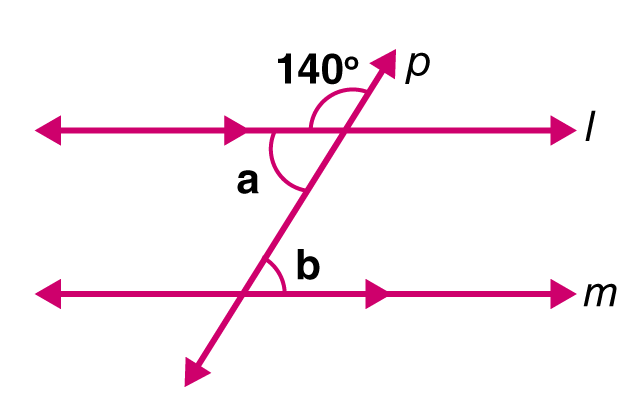(ii)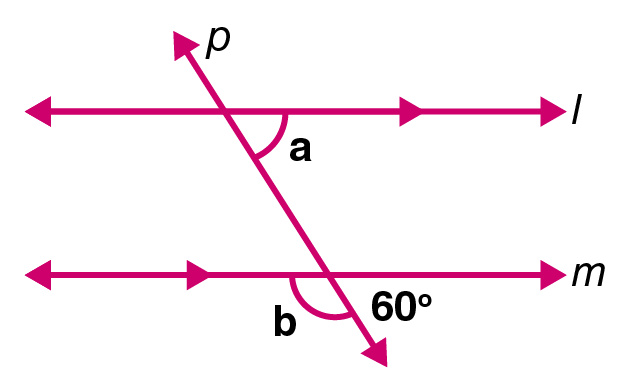(iii)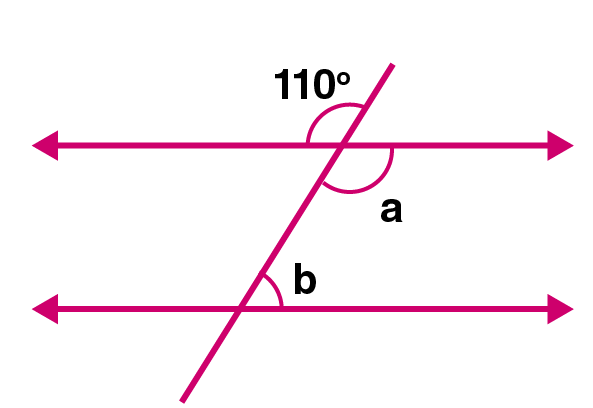(iv)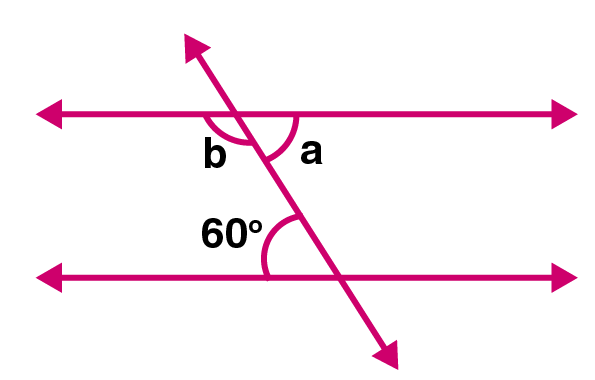(v)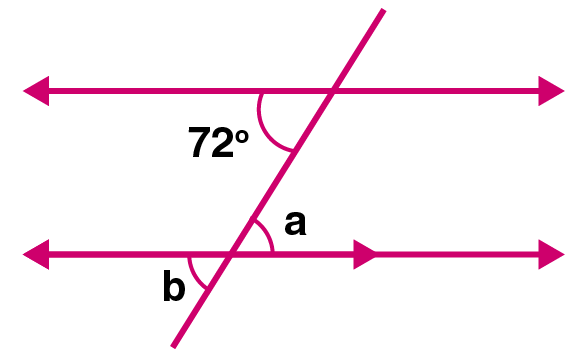Solution:

(i) a + 1400 = 1800 [Linear pair]

a = 1800 – 1400

We get,

a = 400

Here, b = a {alternate angles}

Hence, a = b = 400

(ii) Given

l || m and p intersects them

b + 600 = 1800 [Linear pair]

b = 1800 – 600

We get,

b = 1200

and a = 600 {corresponding angles}

Hence, a = 600

b = 1200

(iii) a = 1100 [Vertically opposite angles]

b = 1800 – a [Co-interior angles]

= 1800 – a

= 1800 – 1100

We get,

= 700

(iv) a = 600 [Alternate interior angles]

b = 1800 – a [Co-interior angles]

= 1800 – 600

We get,

= 1200

(v) a = 720 [Alternate interior angles]

b = a [Vertically opposite angles]

Hence, b = 720

3. If ∠1 = 1200, find the measures of: ∠2, ∠3, ∠4, ∠5, ∠6, ∠7 and ∠8. Give reasons.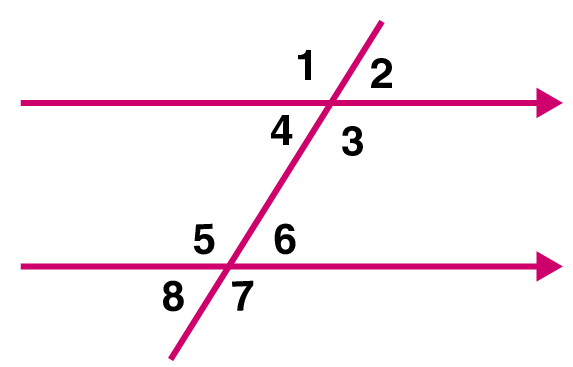Solution:

Given

l || m and p is their transversal and

∠1 = 1200

∠1 + ∠2 = 1800 [Straight line angle]

1200 + ∠2 = 1800

∠2 = 1800 – 1200

We get,

∠2 = 600

Therefore, ∠2 = 600

But ∠1 and ∠3 [Vertically opposite angles]

Hence, ∠3 = ∠1 = 1200

Similarly,

∠4 = ∠2 [Vertically opposite angles]

∠4 = 600

∠5 = ∠1 [Corresponding angles]

Hence, ∠5 = 1200

Similarly,

∠6 = ∠2 [Corresponding angles]

∠6 = 600

∠7 = ∠5 [Vertically opposite angles]

Hence, ∠7 = 1200

and ∠8 = ∠6 [Vertically opposite angles]

Hence, ∠8 = 600

Therefore, the measures of angles are,

∠2 = 600

∠3 = 1200

∠4 = 600

∠5 = 1200

∠6 = 600

∠7 = 1200 and

∠8 = 600

4. In the figure given below, find the measure of the angles denoted by x, y, z, p, q and r.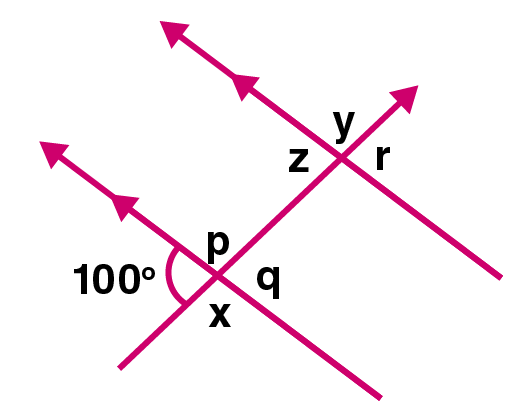Solution:

x = 1800 – 1000 (Linear pair of angles)

x = 800

y = x (Alternate exterior angles)

y = 800

z = 1000 (Corresponding angles)

p = x (Vertically opposite angles)

p = 800

q = 1000 (Vertically opposite angles)

r = q (Corresponding angles)

r = 1000

Therefore, the measures of angles are,

x = y = p = 800

q = r = z = 1000

5. Using the given figure, fill in the blanks.

∠x = …………;

∠z = …………;

∠p = …………;

∠q = …………;

∠r = ………….;

∠s = ………….;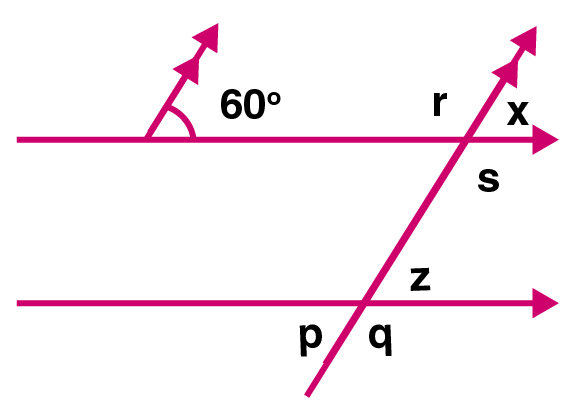Solutions:

∠x = 600 (Corresponding angles)

z = x (Corresponding angles)

= 600

p = z (Vertically opposite angles)

= 600

q = 1800 – p (Linear pair of angles)

= 1800 – 600

We get,

= 1200

r = 1800 – x (Linear pair of angles)

= 1800 – 600

We get,

= 1200

s = r (Vertically opposite angles)

s = r = 1200

6. In the given figure, find the angles shown by x, y, z and w. Give reasons.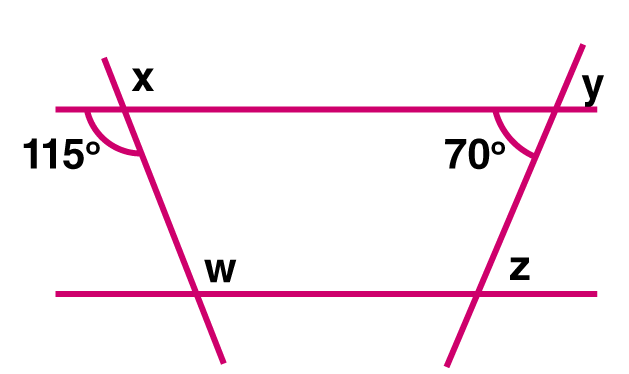Solution:

x = 1150 (By vertically opposite angles)

y = 700 (By vertically opposite angles)

z = 700 (By alternate interior angles)

w = 1150 (By alternate interior angles)

7. Find a, b, c and d in the figure given below: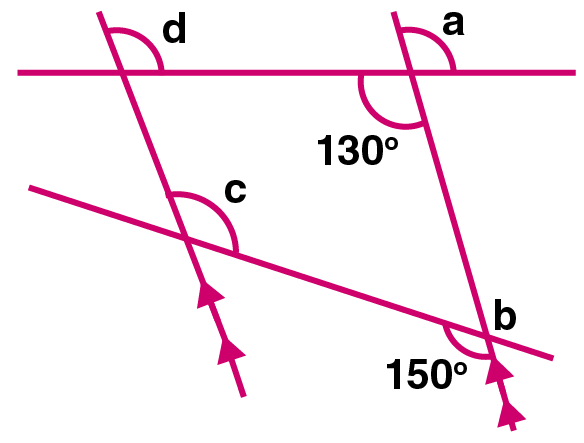Solution:

a = 1300 (By vertically opposite angles)

b = 1500 (By vertically opposite angles)

c = 1500 (By alternate interior angles)

d = 1300 (By alternate interior angles)

8. Find x, y and z in the figure given below: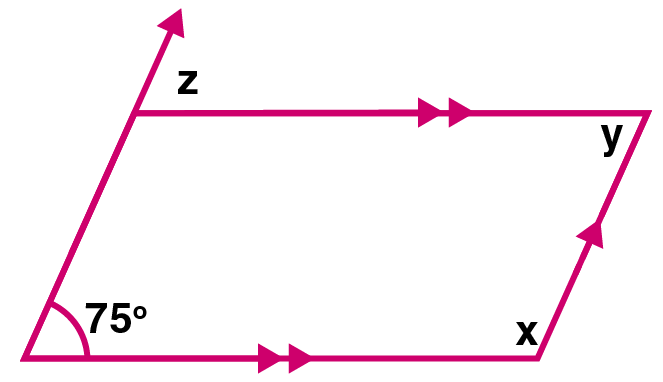Solution:

x = 1800 – 750 (Co-interior angles)

We get,

x = 1050

y = 1800 – x (Co-interior angles)

y = 1800 – 1050

We get,

y = 750

z = 750 (Corresponding angles)

Therefore, the angles are,

x = 1050, y = 750 and z = 750

Exercise 25(C)

1. In your note-book copy the following angles using ruler and a pair compass only.

(i)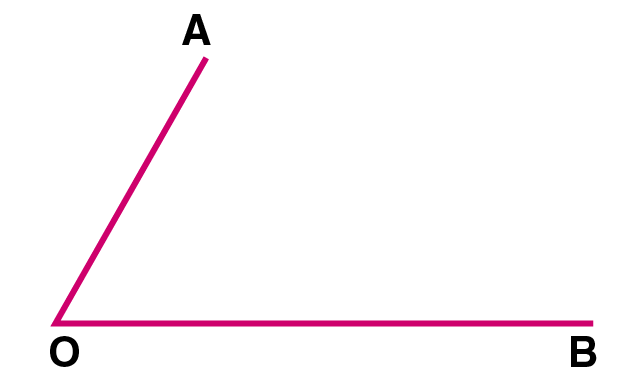(ii)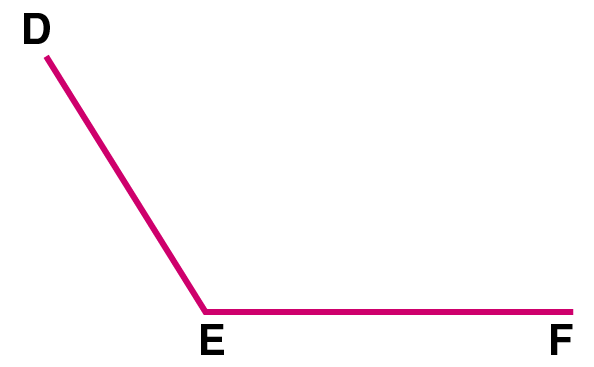(iii)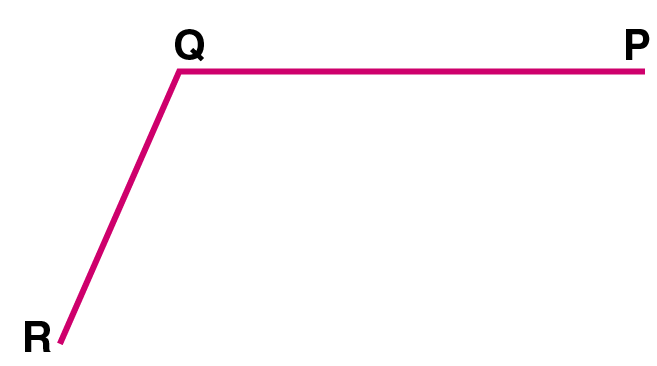Solution:

(i) Steps of Construction:

1. Draw line QR = OB at point Q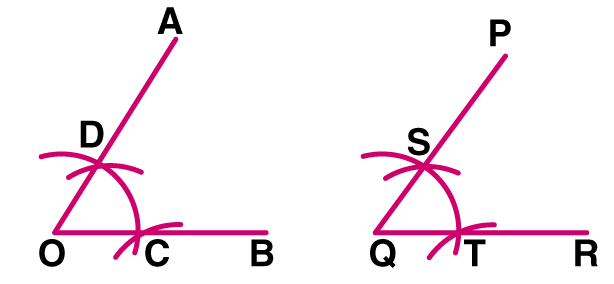2. Keeping O as centre, draw an arc of any suitable radius, to cut the arms of the angle at points C and D

3. Keeping Q as centre, draw the arc of the same size as drawn for C and D. Let this arc line cuts line QR at point T

4. With the help of compasses, take the distance equal to distance between C and D; and then taking T as centre, draw an arc which cuts the earlier arc at point S.

5. Join QS and produce up to a suitable point P. Now, the obtained ∠PQR , is the angle equal to the given ∠AOB

(ii) Steps of Construction:

1. Draw a line EF, at a point E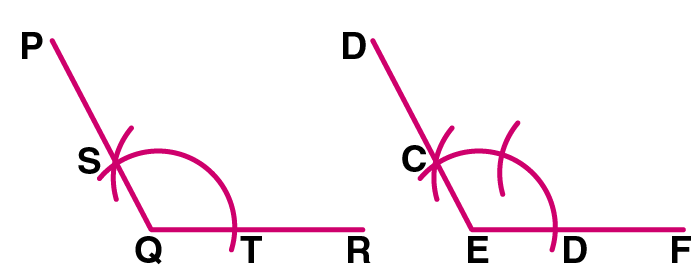2. Taking E as centre, draw an arc of any suitable radius, to cut the arms of the angle at points C and D

3. Taking Q as centre, draw an arc of the same size as drawn for points C and D. Let this arc cuts line QR at point T

4. With the help of compasses, take the distance equal to the distance between C and D; and then taking T as centre, draw an arc which cuts the earlier arc at point S

5. Join QS and produce up to a suitable point S. Now, the obtained ∠PQR is the angle equal to the the given ∠DEF

(iii) Steps of Construction:

1. Draw a line AB = QP at a point A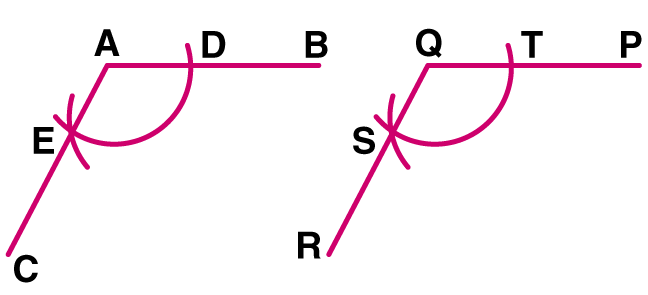2. Taking Q as centre, draw an arc of any suitable radius, to cut the arms of the angle T and S

3. Taking A as centre, draw an arc of the same size as drawn for points T and S. Let this arc cuts the line AB at point D

4. With the help of compasses, take the distance equal to the distance between T and S; and then taking D as centre, draw an arc which cuts the earlier arc at point E

5. Join AE produced up to a suitable point C. The obtained ∠BAC is the angle equal to the given ∠PQR

2. Construct the following angles, using ruler and a pair of compass only

(i) 600

(ii) 900

(iii) 450

(iv) 300

(v) 1200

Solution:

(i) Steps of Construction:

Constructing the angle of 600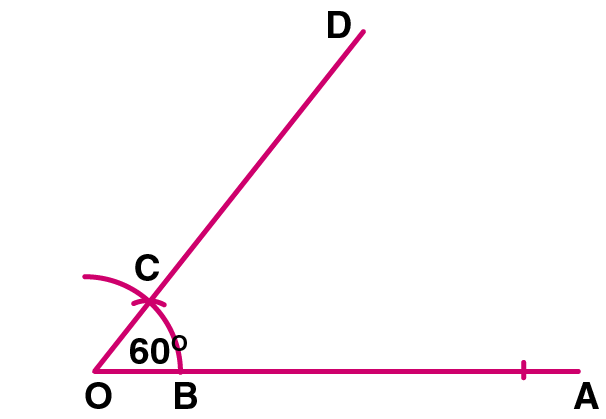1. Draw a line OA of any suitable length

2. Taking O as centre, draw an arc of any size to cut OA at point B

3. Now, taking B as centre, draw the same size arc, to cut the previous arc at point C

4. Join OC and produce up to a suitable point D. Then, the obtained ∠DOA is the angle of 600

(ii) Steps of Construction:

Constructing angle of 900

Let OA be the line and at point O, the angle of 900 is to be drawn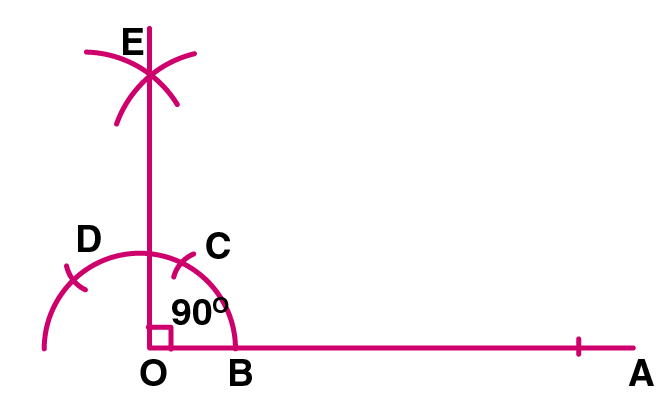1. Taking O as centre, draw an arc to cut the arm OA at point B

2. Taking B as centre, draw the same size arc to cut the previous arc at point C

3. Again with C as centre and with the same radius, draw one more arc to cut the previous arc at point D

4. Now, taking C and D as centres, draw two arcs of equal radii to cut each other at point E.

5. Join O and E. Then ∠AOE = 900 is obtained

(iii) Draw an angle of 900, following the steps as in question (ii) and bisect it. Each angle so obtained will be 450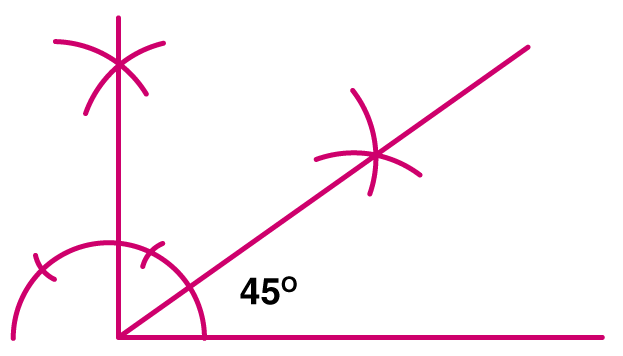(iv) Steps of Construction:

Constructing an angle of 300

1. Draw an angle of 600 following the steps as drawn in question no.(i)

2. Now, bisecting this angle, we get two angles each of 300. Therefore ∠EOB = 300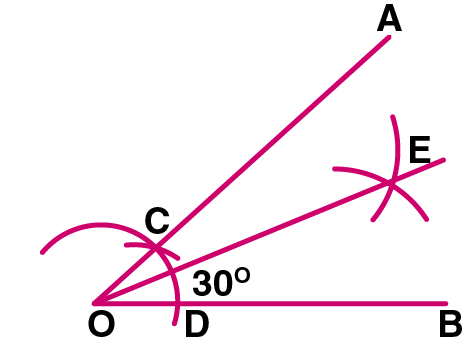(v) Steps of Construction:

Constructing an angle of 1200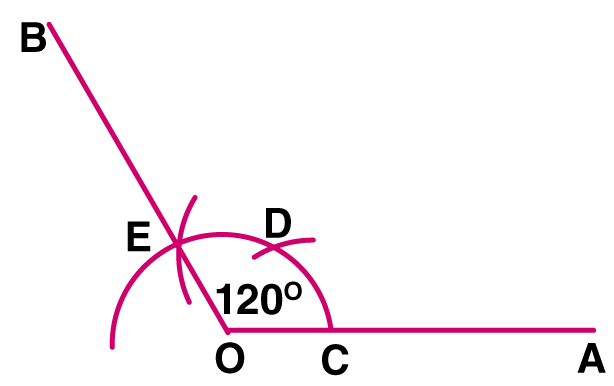1. Taking centre as O on the line OA, draw an arc to cut this line at point C

2. Now, taking C as centre, draw a same size arc which cuts the first arc at point D

3. Taking D as centre, draw one more arc of same size which cuts the first arc at point E

4. Join OE and produce it up to point B. Now, the ∠AOB is the obtained angle whose measure is 1200

3. Draw line AB = 6 cm. Construct angle ABC = 600. Then draw the bisector of angle ABC.

Solution:

Steps of Construction:

1. Draw a line segment AB of length 6 cm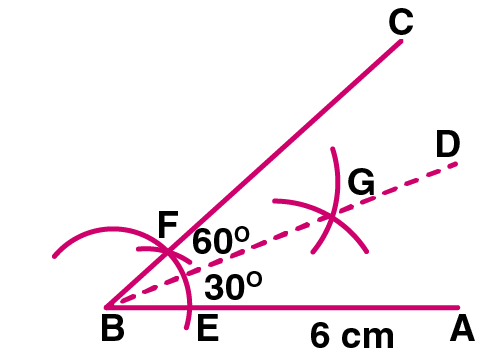2. Using compass construct ∠CBA = 600

3. Bisect ∠CBA, using compass, take any radius which meet line AB and BC at points E and F

4. Now, with the help of compass take radius more than ½ of EF and draw two arcs from point E and F, where both the arcs intersects at point G, proceed BG towards D. Now the ∠DBA is bisector of ∠CBA

4. Draw a line segment PQ = 8 cm. Construct the perpendicular bisector of the line segment PQ. Let the perpendicular bisector drawn meet PQ at point R. Measure the lengths of PR and QR. Is PR = QR?

Solution:

Steps of Construction:

1. Taking P and Q as centres, draw arcs on both sides of PQ with equal radii. The radius should be more than half the length of PQ

2. Let these arcs cut each other at point R and RS

3. Now, join RS which cuts PQ at point D

Now, RS = PQ. Also ∠POR = 900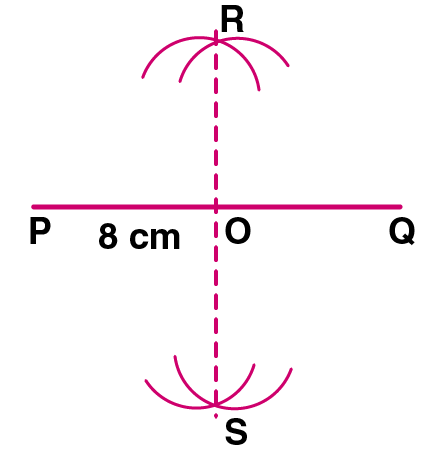Therefore, the line segment RS is the perpendicular bisector of PQ as it bisects PQ at point P and it also perpendicular to PQ. Now, on measuring the length

PR = 4 cm

QR = 4 cm

Since, PR = QR = 4 cm

Therefore, PR = QR

5. Draw a line segment AB = 7 cm. Mark a point AB such that AP = 3 cm. Draw perpendicular on to AB at point P.

Solution:

1. Draw a line segment AB of length 7 cm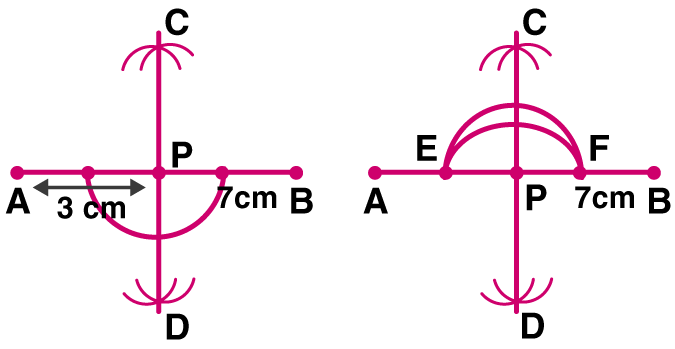2. Mark a point on AB such that, AB – AP = 3 cm

3. From point P, cut arc on outside of AB, and mark them as point E and F

4.Now, from point E and F cut arcs on both side intersecting each other at point C and D

5. Join point P, C and D

6. Which is the required perpendicular

6. Draw a line segment AB = 6.5 cm. Locate a point P that is 5 cm from A and 4.6 cm from B. Through the point P, draw a perpendicular on to the line segment AB.

Solution: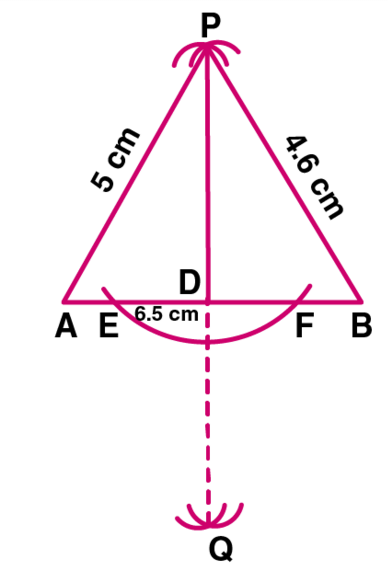Steps of Construction:

(i) Draw a line segment AB of length 6.5 cm

(ii) Taking radius as 5 cm and with centre A, draw an arc and taking radius as 4.6 cm and with centre B, draw another arc which intersects the first arc at point P

Now, P is the required point

(iii) Taking centre A and a suitable radius, draw an arc which intersect AB at points E and F

(iv) Now, taking E and F as centres and radius greater than half of EF, draw the arcs which intersect each other at point Q

(v) Join PQ which intersect AB at point D

(vi) Now, PD is perpendicular to AB

Exercise 25(D)

1. Draw a line segment OA = 5 cm. Use set-square to construct angle AOB = 600, such that OB = 3 cm. Join A and B; then measure the length of AB.

Solution:

The length of AB = 4.4 cm (approximately)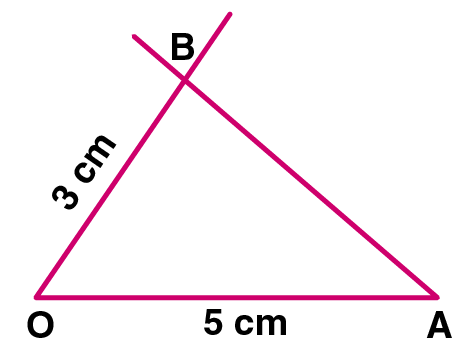2. Draw a line segment OP = 8 cm. Use set-square to construct ∠POQ = 900; such that OQ = 6 cm. Join P and Q; then measure the length of PQ.

Solution: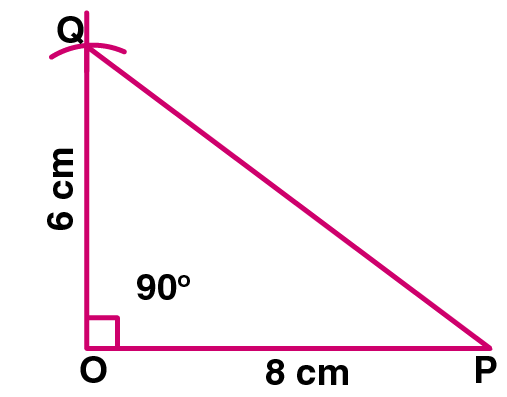Measuring the length of PQ = 10 cm

3. Draw ∠ABC = 1200. Bisect the angle using ruler and compasses. Measure each angle so obtained and check whether or not the new angles obtained on bisecting ∠ABC are equal.

Solution: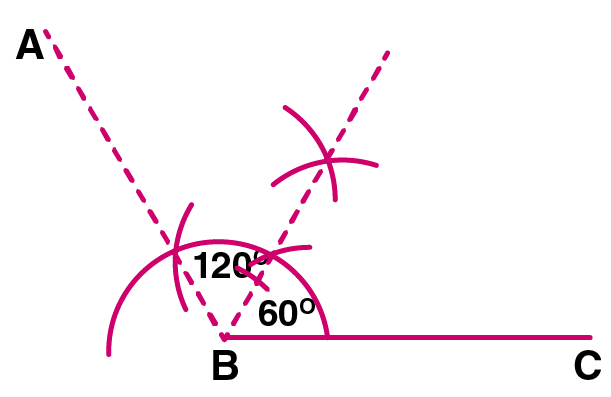Each angle measure = 600

Yes, the angles obtained on bisecting ∠ABC are equal

4. Draw ∠PQR = 750 by using set-squares. On PQ mark a point M such that MQ = 3 cm. On QR mark a point N such that QN = 4 cm. Join M and N. Measure the length of MN.

Solution: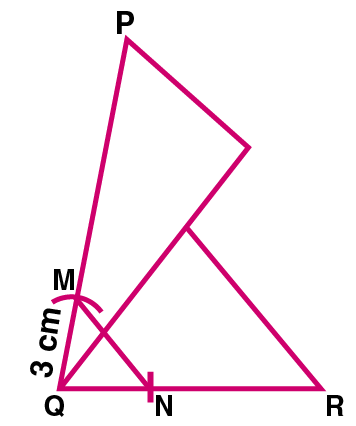The length of MN = 4.3 cm